Courses

# Test: Logical Sequence- 1

## 10 Questions MCQ Test Quantitative Aptitude for Banking Preparation | Test: Logical Sequence- 1

Description
This mock test of Test: Logical Sequence- 1 for UPSC helps you for every UPSC entrance exam. This contains 10 Multiple Choice Questions for UPSC Test: Logical Sequence- 1 (mcq) to study with solutions a complete question bank. The solved questions answers in this Test: Logical Sequence- 1 quiz give you a good mix of easy questions and tough questions. UPSC students definitely take this Test: Logical Sequence- 1 exercise for a better result in the exam. You can find other Test: Logical Sequence- 1 extra questions, long questions & short questions for UPSC on EduRev as well by searching above.
QUESTION: 1

### I wear a pad every time I bat. (i) I batted. (ii) I didn’t bat. (iii) I wore a pad. (iv) I didn’t wear a pad.

Solution:

If I wear a pad every time I bat, then it also shows that if I did not wear a pad, I did not bat. So, (ii) follows (iv) logically.
Please note that wearing a pad does not mean I batted. Logic is given other way round, that If I bat, then I wear a pad.
I may wear a pad, but do not bat. Correct option is (d)

QUESTION: 2

### A poem has seven lines, each of which starts with the letters P, Q, R, S, T, U and V. The line starting with R is the third from the bottom and the line starting with V is the second from the top. The line starting with Q and that starting with U have exactly three lines in between. Either S or P is the only letter between R and U. The poem is likely to start with

Solution:

It is given that R is 3rd from the bottom and V is 2nd from the top, then R and U have only one line between them, i.e. either S or P. Now the possible arrangement will be as Follows.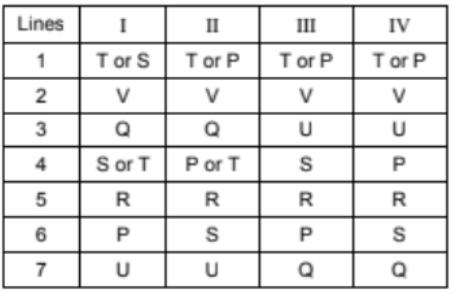So, T or S or P has to be in line 1 and the poem starts with T or S or P.

QUESTION: 3

### K, L, M, N, O and P are six trees in a garden. Neither M nor N is the tallest tree. Tree L is taller than P but shorter than tree K. Tree O is as tall as P but taller than M. Which of the following trees is the tallest?

Solution:

Neither tree M nor tree N is the tallest.
Tree L is taller than tree P but is shorter than tree K,
whereas tree O is as tall as tree P, but taller than tree M.
So, the order may be as follows
1    2    3   4
K    L   P   M
-     -    O   -
As the tree N is not the tallest, K must be the tallest. Choice (b)

QUESTION: 4

I will buy the airplane only if it is the costliest and the fastest.

Solution:

If I bought aeroplane, it would be the fastest & the costliest. If I did not buy the aeroplane it is either not the fastest or not the costliest or neither fastest nor the costliest (not necessarily neither fastest nor the costliest). Only option (c) show a feasible case of buying the aeroplane

QUESTION: 5

Six persons A, B, C, D, E and F leave for the station in such a way that C leaves before F leaves and after D leaves, whereas E leaves before B leaves, who leaves before F leaves, but A leaves after both F and C leave. Who is the last person to leave?

Solution:

As it is given that C leaves the station before F leaves, but after D leaves, i.e. D > C > F.
So D C F can be the order of their departure.
E leaves the station before B leaves, who leaves before
F leaves, i.e. E > B > F.
So E B F can be the order of their departure.
A leaves the station after F and C leave the station,
i.e. C > F> A.
So A is the last person to leave as shown below.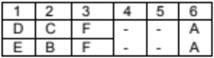QUESTION: 6

If the milk is not cold then I will not go to school and will not have dinner.

Solution:

If the milk is not cold then I will not go to the school & will not have dinner. Then, if I had gone to school or I had dinner, the milk is cold. Correct option is (c)

QUESTION: 7

These questions are based on the following information.
P, Q, R are three girls and A, B, C are three boys.
Q is taller than R but shorter than C, who is taller than A.
P is taller than B, who is taller than C.

Who is the tallest of all the six?

Solution:

The given conditions are as follows.
P, Q, R are three girls.
A, B, C are three boys.
Q is taller than R i.e. Q > R.
C is taller than Q i.e. C > Q.
C is taller than A i.e. C > A.
P is taller than B i.e. P > B.
B is taller than C i.e. B > C.
Now, the arrangement can be as follows.
C > Q > R and P > B > C and C > R.
Now, after considering all the above given conditions, the
The arrangement is as follows.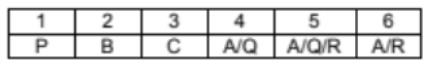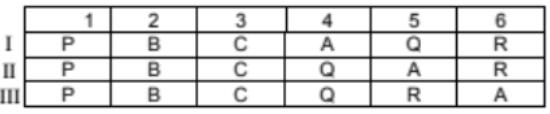P is the tallest of all.

QUESTION: 8

These questions are based on the following information.
P, Q, R are three girls and A, B, C are three boys.
Q is taller than R but shorter than C, who is taller than A.
P is taller than B, who is taller than C.

Who is the shortest of the three girls?

Solution:

The given conditions are as follows.
P, Q, R are three girls.
A, B, C are three boys.
Q is taller than R i.e. Q > R.
C is taller than Q i.e. C > Q.
C is taller than A i.e. C > A.
P is taller than B i.e. P > B.
B is taller than C i.e. B > C.
Now, the arrangement can be as follows.
C > Q > R and P > B > C and C > R.
Now, after considering all the above given conditions, the
The arrangement is as follows.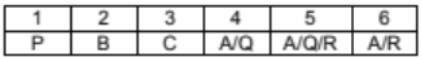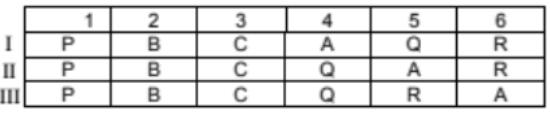R is the shortest among the three girls. P > Q > R.

QUESTION: 9

You cannot clear the CAT unless you are hard working.
(i) You are hard working.
(ii) You can clear the CAT.
(iii) You are not hard working.
(iv) You cannot clear the CAT.

Solution:

If you cannot clear the CAT unless you are hardworking, then if you are not hardworking you cannot clear the CAT. So, (iv) follows (iii) logically Correct option is (d)

QUESTION: 10

Whenever my mother scolds me, I either hide behind my father or complain to my grandma.

Solution:

If whenever my mother scolds me, I either hide behind my father or complain to my grandma. Then, if I did not complain to my grandma & did not hide behind my father, my mother must not have scolded me. Correct option is (a)

Track your progress, build streaks, highlight & save important lessons and more!

### Similar Content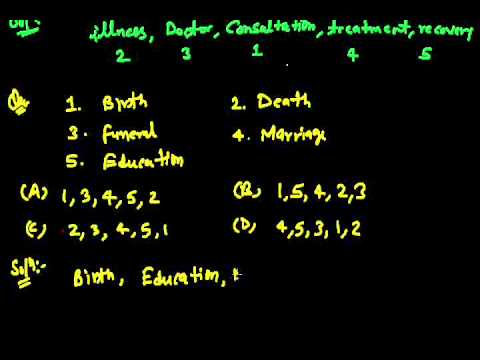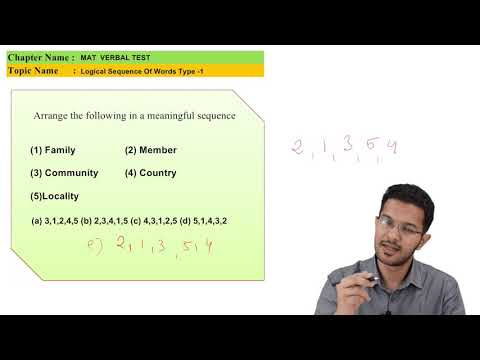### Related tests